Anzeige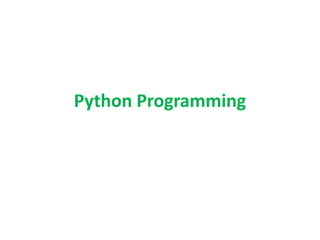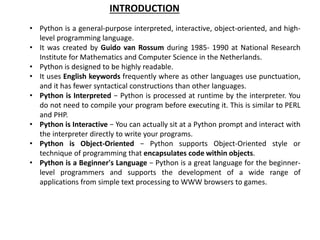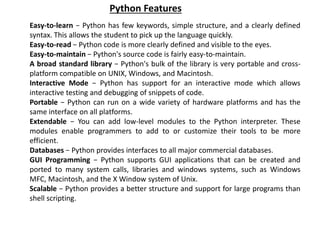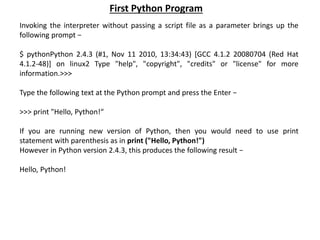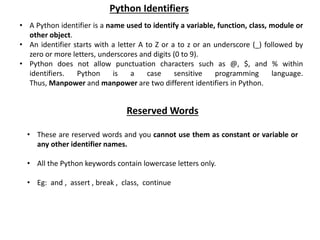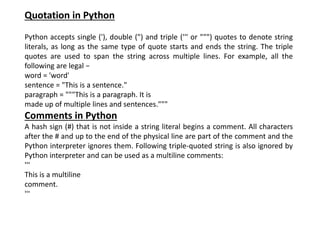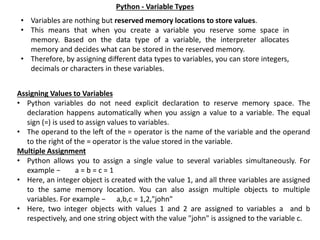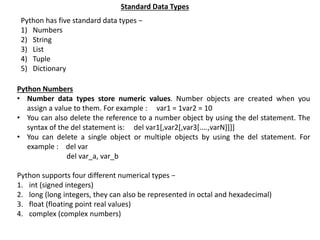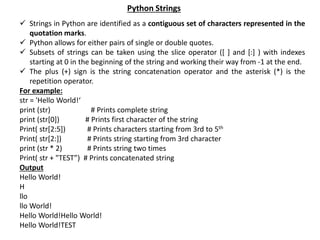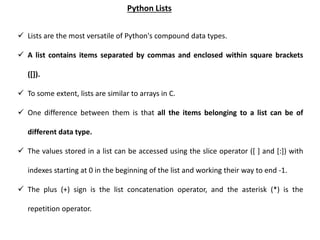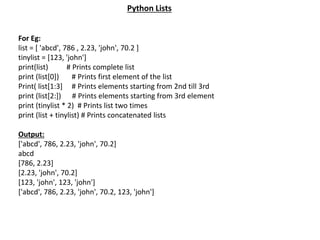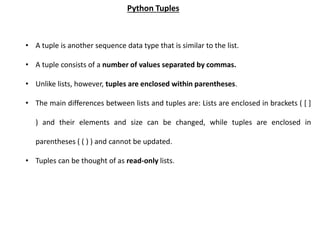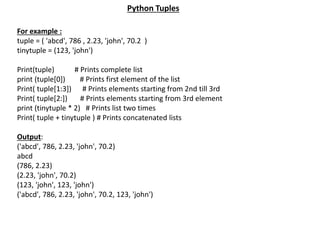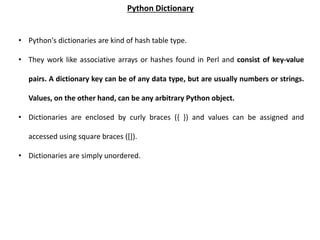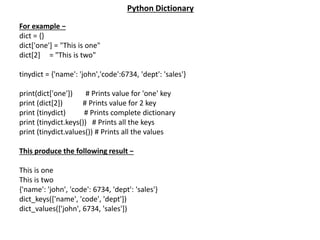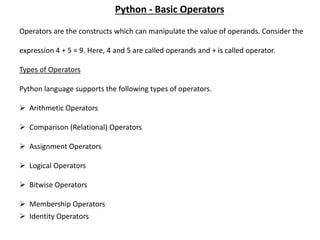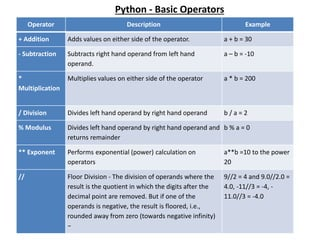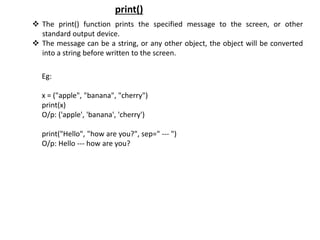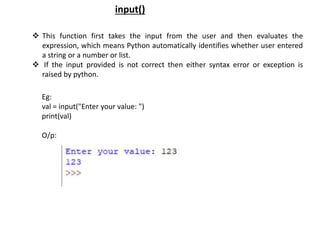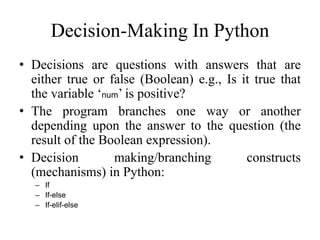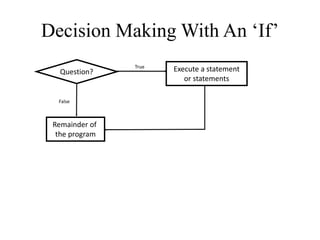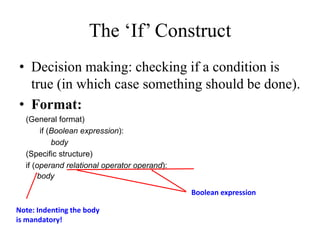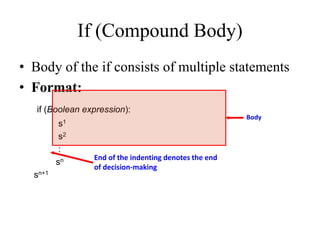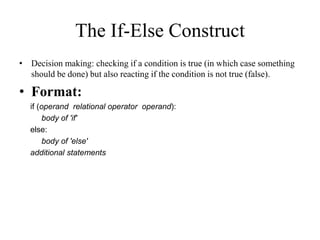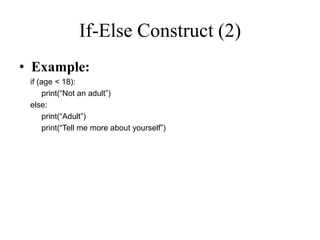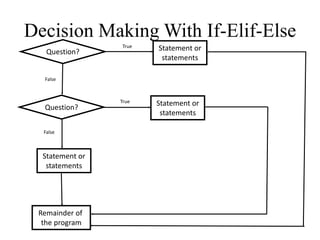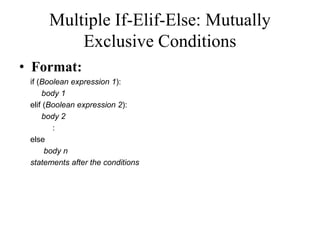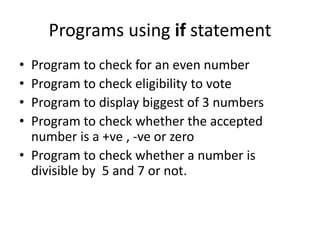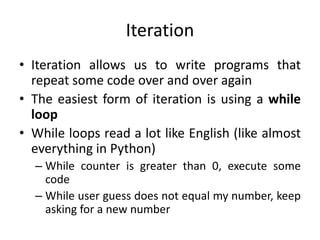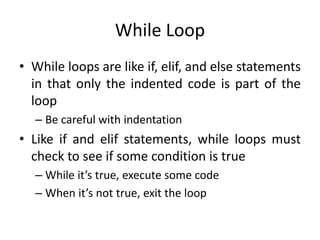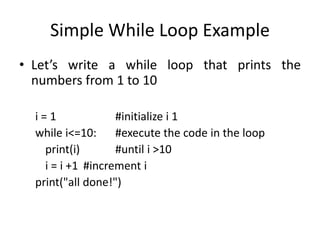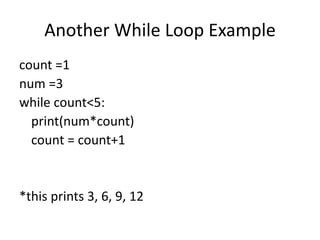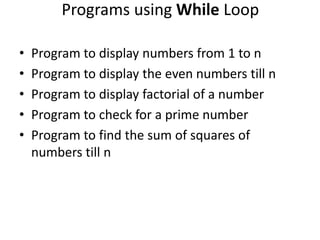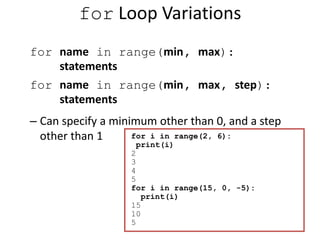1 von 35
Anzeige

### 1. python programming

1. Python Programming
2. • Python is a general-purpose interpreted, interactive, object-oriented, and high- level programming language. • It was created by Guido van Rossum during 1985- 1990 at National Research Institute for Mathematics and Computer Science in the Netherlands. • Python is designed to be highly readable. • It uses English keywords frequently where as other languages use punctuation, and it has fewer syntactical constructions than other languages. • Python is Interpreted − Python is processed at runtime by the interpreter. You do not need to compile your program before executing it. This is similar to PERL and PHP. • Python is Interactive − You can actually sit at a Python prompt and interact with the interpreter directly to write your programs. • Python is Object-Oriented − Python supports Object-Oriented style or technique of programming that encapsulates code within objects. • Python is a Beginner's Language − Python is a great language for the beginner- level programmers and supports the development of a wide range of applications from simple text processing to WWW browsers to games. INTRODUCTION
3. Easy-to-learn − Python has few keywords, simple structure, and a clearly defined syntax. This allows the student to pick up the language quickly. Easy-to-read − Python code is more clearly defined and visible to the eyes. Easy-to-maintain − Python's source code is fairly easy-to-maintain. A broad standard library − Python's bulk of the library is very portable and cross- platform compatible on UNIX, Windows, and Macintosh. Interactive Mode − Python has support for an interactive mode which allows interactive testing and debugging of snippets of code. Portable − Python can run on a wide variety of hardware platforms and has the same interface on all platforms. Extendable − You can add low-level modules to the Python interpreter. These modules enable programmers to add to or customize their tools to be more efficient. Databases − Python provides interfaces to all major commercial databases. GUI Programming − Python supports GUI applications that can be created and ported to many system calls, libraries and windows systems, such as Windows MFC, Macintosh, and the X Window system of Unix. Scalable − Python provides a better structure and support for large programs than shell scripting. Python Features
4. Invoking the interpreter without passing a script file as a parameter brings up the following prompt − \$ pythonPython 2.4.3 (#1, Nov 11 2010, 13:34:43) [GCC 4.1.2 20080704 (Red Hat 4.1.2-48)] on linux2 Type "help", "copyright", "credits" or "license" for more information.>>> Type the following text at the Python prompt and press the Enter − >>> print "Hello, Python!“ If you are running new version of Python, then you would need to use print statement with parenthesis as in print ("Hello, Python!") However in Python version 2.4.3, this produces the following result − Hello, Python! First Python Program
5. • A Python identifier is a name used to identify a variable, function, class, module or other object. • An identifier starts with a letter A to Z or a to z or an underscore (_) followed by zero or more letters, underscores and digits (0 to 9). • Python does not allow punctuation characters such as @, \$, and % within identifiers. Python is a case sensitive programming language. Thus, Manpower and manpower are two different identifiers in Python. Python Identifiers Reserved Words • These are reserved words and you cannot use them as constant or variable or any other identifier names. • All the Python keywords contain lowercase letters only. • Eg: and , assert , break , class, continue
6. Quotation in Python Python accepts single ('), double (") and triple (''' or """) quotes to denote string literals, as long as the same type of quote starts and ends the string. The triple quotes are used to span the string across multiple lines. For example, all the following are legal − word = 'word' sentence = "This is a sentence." paragraph = """This is a paragraph. It is made up of multiple lines and sentences.""" Comments in Python A hash sign (#) that is not inside a string literal begins a comment. All characters after the # and up to the end of the physical line are part of the comment and the Python interpreter ignores them. Following triple-quoted string is also ignored by Python interpreter and can be used as a multiline comments: ''' This is a multiline comment. '''
7. Python - Variable Types • Variables are nothing but reserved memory locations to store values. • This means that when you create a variable you reserve some space in memory. Based on the data type of a variable, the interpreter allocates memory and decides what can be stored in the reserved memory. • Therefore, by assigning different data types to variables, you can store integers, decimals or characters in these variables. Assigning Values to Variables • Python variables do not need explicit declaration to reserve memory space. The declaration happens automatically when you assign a value to a variable. The equal sign (=) is used to assign values to variables. • The operand to the left of the = operator is the name of the variable and the operand to the right of the = operator is the value stored in the variable. Multiple Assignment • Python allows you to assign a single value to several variables simultaneously. For example − a = b = c = 1 • Here, an integer object is created with the value 1, and all three variables are assigned to the same memory location. You can also assign multiple objects to multiple variables. For example − a,b,c = 1,2,"john" • Here, two integer objects with values 1 and 2 are assigned to variables a and b respectively, and one string object with the value "john" is assigned to the variable c.
8. Standard Data Types Python has five standard data types − 1) Numbers 2) String 3) List 4) Tuple 5) Dictionary Python Numbers • Number data types store numeric values. Number objects are created when you assign a value to them. For example : var1 = 1var2 = 10 • You can also delete the reference to a number object by using the del statement. The syntax of the del statement is: del var1[,var2[,var3[....,varN]]]] • You can delete a single object or multiple objects by using the del statement. For example : del var del var_a, var_b Python supports four different numerical types − 1. int (signed integers) 2. long (long integers, they can also be represented in octal and hexadecimal) 3. float (floating point real values) 4. complex (complex numbers)
9.  Strings in Python are identified as a contiguous set of characters represented in the quotation marks.  Python allows for either pairs of single or double quotes.  Subsets of strings can be taken using the slice operator ([ ] and [:] ) with indexes starting at 0 in the beginning of the string and working their way from -1 at the end.  The plus (+) sign is the string concatenation operator and the asterisk (*) is the repetition operator. For example: str = 'Hello World!‘ print (str) # Prints complete string print (str) # Prints first character of the string Print( str[2:5]) # Prints characters starting from 3rd to 5th Print( str[2:]) # Prints string starting from 3rd character print (str * 2) # Prints string two times Print( str + "TEST”) # Prints concatenated string Output Hello World! H llo llo World! Hello World!Hello World! Hello World!TEST Python Strings
10.  Lists are the most versatile of Python's compound data types.  A list contains items separated by commas and enclosed within square brackets ([]).  To some extent, lists are similar to arrays in C.  One difference between them is that all the items belonging to a list can be of different data type.  The values stored in a list can be accessed using the slice operator ([ ] and [:]) with indexes starting at 0 in the beginning of the list and working their way to end -1.  The plus (+) sign is the list concatenation operator, and the asterisk (*) is the repetition operator. Python Lists
11. For Eg: list = [ 'abcd', 786 , 2.23, 'john', 70.2 ] tinylist = [123, 'john'] print(list) # Prints complete list print (list) # Prints first element of the list Print( list[1:3] # Prints elements starting from 2nd till 3rd print (list[2:]) # Prints elements starting from 3rd element print (tinylist * 2) # Prints list two times print (list + tinylist) # Prints concatenated lists Output: ['abcd', 786, 2.23, 'john', 70.2] abcd [786, 2.23] [2.23, 'john', 70.2] [123, 'john', 123, 'john'] ['abcd', 786, 2.23, 'john', 70.2, 123, 'john'] Python Lists
12. • A tuple is another sequence data type that is similar to the list. • A tuple consists of a number of values separated by commas. • Unlike lists, however, tuples are enclosed within parentheses. • The main differences between lists and tuples are: Lists are enclosed in brackets ( [ ] ) and their elements and size can be changed, while tuples are enclosed in parentheses ( ( ) ) and cannot be updated. • Tuples can be thought of as read-only lists. Python Tuples
13. For example : tuple = ( 'abcd', 786 , 2.23, 'john', 70.2 ) tinytuple = (123, 'john') Print(tuple) # Prints complete list print (tuple) # Prints first element of the list Print( tuple[1:3]) # Prints elements starting from 2nd till 3rd Print( tuple[2:]) # Prints elements starting from 3rd element print (tinytuple * 2) # Prints list two times Print( tuple + tinytuple ) # Prints concatenated lists Output: ('abcd', 786, 2.23, 'john', 70.2) abcd (786, 2.23) (2.23, 'john', 70.2) (123, 'john', 123, 'john') ('abcd', 786, 2.23, 'john', 70.2, 123, 'john') Python Tuples
14. • Python's dictionaries are kind of hash table type. • They work like associative arrays or hashes found in Perl and consist of key-value pairs. A dictionary key can be of any data type, but are usually numbers or strings. Values, on the other hand, can be any arbitrary Python object. • Dictionaries are enclosed by curly braces ({ }) and values can be assigned and accessed using square braces ([]). • Dictionaries are simply unordered. Python Dictionary
15. For example − dict = {} dict['one'] = "This is one" dict = "This is two" tinydict = {'name': 'john','code':6734, 'dept': 'sales'} print(dict['one']) # Prints value for 'one' key print (dict) # Prints value for 2 key print (tinydict) # Prints complete dictionary print (tinydict.keys()) # Prints all the keys print (tinydict.values()) # Prints all the values This produce the following result − This is one This is two {'name': 'john', 'code': 6734, 'dept': 'sales'} dict_keys(['name', 'code', 'dept']) dict_values(['john', 6734, 'sales']) Python Dictionary
16. Operators are the constructs which can manipulate the value of operands. Consider the expression 4 + 5 = 9. Here, 4 and 5 are called operands and + is called operator. Types of Operators Python language supports the following types of operators.  Arithmetic Operators  Comparison (Relational) Operators  Assignment Operators  Logical Operators  Bitwise Operators  Membership Operators  Identity Operators Python - Basic Operators
17. Python - Basic Operators Operator Description Example + Addition Adds values on either side of the operator. a + b = 30 - Subtraction Subtracts right hand operand from left hand operand. a – b = -10 * Multiplication Multiplies values on either side of the operator a * b = 200 / Division Divides left hand operand by right hand operand b / a = 2 % Modulus Divides left hand operand by right hand operand and returns remainder b % a = 0 ** Exponent Performs exponential (power) calculation on operators a**b =10 to the power 20 // Floor Division - The division of operands where the result is the quotient in which the digits after the decimal point are removed. But if one of the operands is negative, the result is floored, i.e., rounded away from zero (towards negative infinity) − 9//2 = 4 and 9.0//2.0 = 4.0, -11//3 = -4, - 11.0//3 = -4.0
18.  The print() function prints the specified message to the screen, or other standard output device.  The message can be a string, or any other object, the object will be converted into a string before written to the screen. print() Eg: x = ("apple", "banana", "cherry") print(x) O/p: ('apple', 'banana', 'cherry') print("Hello", "how are you?", sep=" --- ") O/p: Hello --- how are you?
19.  This function first takes the input from the user and then evaluates the expression, which means Python automatically identifies whether user entered a string or a number or list.  If the input provided is not correct then either syntax error or exception is raised by python. input() Eg: val = input("Enter your value: ") print(val) O/p:
20. Decision-Making In Python • Decisions are questions with answers that are either true or false (Boolean) e.g., Is it true that the variable ‘num’ is positive? • The program branches one way or another depending upon the answer to the question (the result of the Boolean expression). • Decision making/branching constructs (mechanisms) in Python: – If – If-else – If-elif-else
21. Decision Making With An ‘If’ Question? Execute a statement or statements True False Remainder of the program
22. The ‘If’ Construct • Decision making: checking if a condition is true (in which case something should be done). • Format: (General format) if (Boolean expression): body (Specific structure) if (operand relational operator operand): body Boolean expression Note: Indenting the body is mandatory!
23. • Body of the if consists of multiple statements • Format: if (Boolean expression): s1 s2 : sn sn+1 Body If (Compound Body) End of the indenting denotes the end of decision-making
24. • Decision making: checking if a condition is true (in which case something should be done) but also reacting if the condition is not true (false). • Format: if (operand relational operator operand): body of 'if' else: body of 'else' additional statements The If-Else Construct
25. If-Else Construct (2) • Example: if (age < 18): print(“Not an adult”) else: print(“Adult”) print(“Tell me more about yourself”)
26. Decision Making With If-Elif-Else Question? True Statement or statements False Question? Remainder of the program Statement or statements False True Statement or statements
27. Multiple If-Elif-Else: Mutually Exclusive Conditions • Format: if (Boolean expression 1): body 1 elif (Boolean expression 2): body 2 : else body n statements after the conditions
28. Programs using if statement • Program to check for an even number • Program to check eligibility to vote • Program to display biggest of 3 numbers • Program to check whether the accepted number is a +ve , -ve or zero • Program to check whether a number is divisible by 5 and 7 or not.
29. Iteration • Iteration allows us to write programs that repeat some code over and over again • The easiest form of iteration is using a while loop • While loops read a lot like English (like almost everything in Python) – While counter is greater than 0, execute some code – While user guess does not equal my number, keep asking for a new number
30. While Loop • While loops are like if, elif, and else statements in that only the indented code is part of the loop – Be careful with indentation • Like if and elif statements, while loops must check to see if some condition is true – While it’s true, execute some code – When it’s not true, exit the loop
31. Simple While Loop Example • Let’s write a while loop that prints the numbers from 1 to 10 i = 1 #initialize i 1 while i<=10: #execute the code in the loop print(i) #until i >10 i = i +1 #increment i print("all done!")
32. Another While Loop Example count =1 num =3 while count<5: print(num*count) count = count+1 *this prints 3, 6, 9, 12
33. Programs using While Loop • Program to display numbers from 1 to n • Program to display the even numbers till n • Program to display factorial of a number • Program to check for a prime number • Program to find the sum of squares of numbers till n
34. The for Loop for name in range(max): statements – Repeats for values 0 (inclusive) to max (exclusive) for i in range(5): print(i) 0 1 2 3 4
35. for Loop Variations for name in range(min, max): statements for name in range(min, max, step): statements – Can specify a minimum other than 0, and a step other than 1 for i in range(2, 6): print(i) 2 3 4 5 for i in range(15, 0, -5): print(i) 15 10 5
Anzeige